# Non Verbal Reasoning - Image Analysis

Exercise : Image Analysis - Section 1
1.

Find out which of the figures (1), (2), (3) and (4) can be formed from the pieces given in figure (X).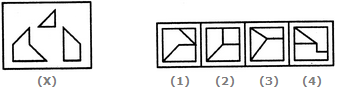1
2
3
4
Explanation:
No answer description is available. Let's discuss.

2.

Find out which of the figures (1), (2), (3) and (4) can be formed from the pieces given in figure (X).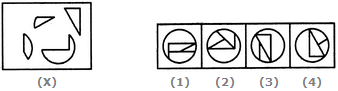1
2
3
4
Explanation:
No answer description is available. Let's discuss.

3.

Find out which of the figures (1), (2), (3) and (4) can be formed from the pieces given in figure (X).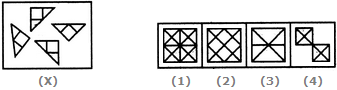1
2
3
4
Explanation:
No answer description is available. Let's discuss.

4.

Find out which of the figures (1), (2), (3) and (4) can be formed from the pieces given in figure (X).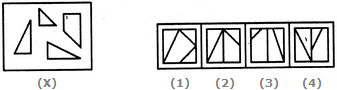1
2
3
4
Explanation:
No answer description is available. Let's discuss.

5.

Find out which of the figures (1), (2), (3) and (4) can be formed from the pieces given in figure (X).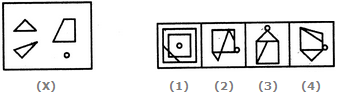1
2
3
4